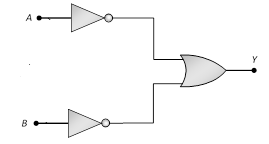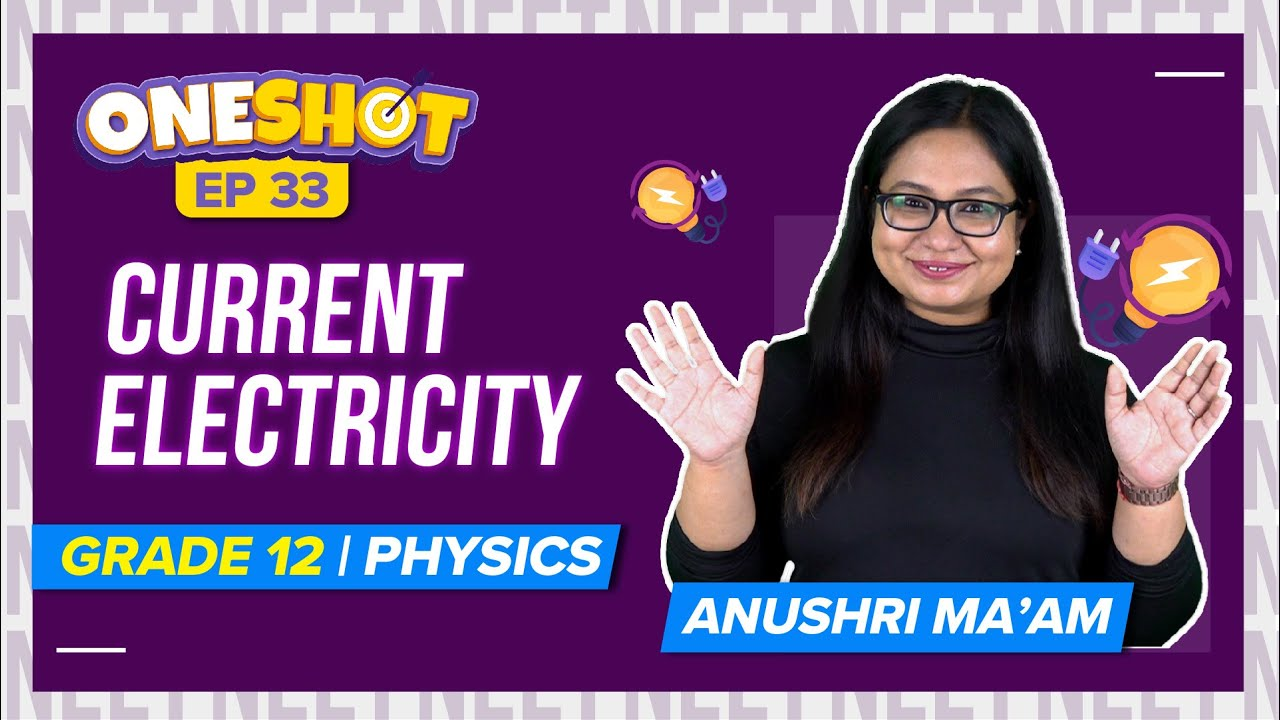Win up to 100% scholarship on Aakash BYJU'S JEE/NEET courses with ABNAT Win up to 100% scholarship on Aakash BYJU'S JEE/NEET courses with ABNAT

# Digital Electronics MCQs For NEET

Digital electronics is the field of physics that deals with digital signals and the devices that produce or use these signals. The digital circuits include diodes and resistors which are used to control the current and voltage in the circuit. Many devices that we see around us like a laptop, televisions, remote control, etc make use of digital electronics.

Q1: The following truth table corresponds to the logic gate

 Input Output A B X 0 0 0 0 1 1 1 1 1 1 0 1
1. NAND
2. OR
3. AND
4. XOR

Q2: Boolean Algebra is essentially based on

1. Truth
2. Logic
3. Symbol
4. Numbers

Q3: The logic behind NOR gate is that it gives

1. HIgh output when both the inputs are low
2. Low output when both the inputs are low
3. HIgh output when both the inputs are high
4. None of the above

Answer: (a) HIgh output when both the inputs are low

Q4: A logic gate is an electronic circuit which

1. Makes logic decisions
2. Allows electrons flow only in one direction
3. Woks binary algebra
4. Alternates between 0 and 1 values

Q5: How many NAND gates are used to form an AND gate?

1. 1
2. 2
3. 3
4. 4

Q6: Which logic gate is represented by the following combination of logic gates?1. OR
2. NAND
3. AND
4. NOR

Q7: The output of OR gate is 1

1. If both inputs are zero
2. If either or both inputs are 1
3. Only if both inputs are 1
4. If either input is zero

Answer: (b) If either or both inputs are 1

Q8: Which of the following logic gate is a universal gate?

1. OR
2. NOT
3. AND
4. NOR

Q9: A gate in which all the inputs must be low to get a high output is

1. NAND gate
2. Inverter
3. NOR gate
4. AND gate

Answer: (b) and (c) Inverter and NOR gate

Q10: The outputs of a NAND gate is 0

1. If both inputs are 0
2. If one input is 0 and the other input is 1
3. If both inputs are 1
4. If both inputs are 1 or one of the inputs is 1 and the other one is 0

Answer: (c) If both inputs are 1

Related NEET Physics MCQs:

### Recommended Video:

Current Electricity Class 12 Physics | NEET 2023 Exam